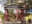Trending ▼   ResFinder# CBSE Pre Board Class 10 2020 : Mathematics (Gokhale Memorial Girls School, Kolkata)

5 pages, 57 questions, 1 questions with responses, 1 total responses,20Sumanta Pan Oxford High School, Howrah
+Fave Message
 Home > sumanta4evr >   F Also featured on: School Page cbse10_pre_boards and 2 moreFormatting page ...

Gokhale Memorial Girls' School CLASS X (2019-20) MATHEMATICS STANDARD (041) Time : 3 Hours Maximum Marks : 80 General Instructions : (i) (ii) (iii) (iv) (v) All questions are compulsory. The questions paper consists of 40 questions divided into four sections A, B, C and D. Section A comprises of 20 questions of 1 mark each. Section B comprises of 6 questions of 2 marks each. Section C comprises of 8 questions of 3 marks each. Section D comprises of 6 questions of 4 marks each. There is no overall choice. However, an internal choices have been provided in two questions of 1 mark each, two questions of 2 marks each, three questions of 3 marks each, and three questions of 4 marks each. You have to attempt only one of the alternatives in all such questions. Use of calculators is not permitted. SECTION A Q.1-Q.10 are multiple choice questions. Select the most appropriate answer from the given options. Q1. Q2. Q3. Q4. Q5. Q6. Q7. Q8. 1 2 If p1 and p2 are two odd prime numbers such that p1 > p2, then p 2 p 2 is (a) an even number (b) an odd number (c) an odd prime number (d) a prime number The points (7, 2) and ( 1, 0) lie on a line 1 is a root of the equation x2 + kx 5 = 0, then the value of k is If (a) 7y = 3x 7 (b) 4y = x + 1 2 4 (c) y = 7x + 7 (d) x = 4y + 1 (a) 2 (b) 2 1 (c) (d) 1 4 2 If the nth term of an A.P. is given by an = 5n 3, then the sum of first 10 terms if (a) 225 (b) 245 (c) 255 (d) 270 ar(TPRQ) It is given that TABC + TPQR with BC = 1 . Then is equal to 3 QR ar(TBCA) (a) 9 (b) 3 (c) 1 (d) 1 3 9 Ratio in which the line 3x + 4y = 7 divides the line joining the points (1, 2) and ( 2, 1) is (a) 3 : 5 (b) 4 segment :6 (c) 4 : 9 (d) None of these (cos4A sin4A) is equal to        (a) 1 2 cos2A (b) 2 sin2A 1 (c) sin2A cos2A (d) 2 cos2A 1 Two chords AB and CD of a circle intersect at E such that AE = 2.4 cm, BE = 3.2 cm and CE = 1.6 cm. The length of DE is (a) 1.6 cm (b) 3.2 cm (c) 4.8 cm (d) 6.4 cm  Page 1Formatting page ...

Top Contributors
to this ResPaperResPaper Admins(1)Sumanta Pan(1)Formatting page ...Formatting page ...Formatting page ...